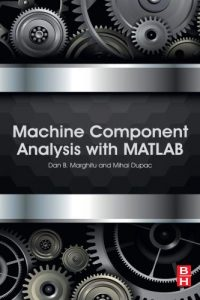اسم المؤلف
Dan B. Marghitu and Mihai Dupac
التاريخ
17 يناير 2021
المشاهدات
التقييم(لا توجد تقييمات)Loading...

Machine Component Analysis with MATLAB
By Dan B. Marghitu and Mihai Dupac
Contents
Preface vii
1. Stress and deflection 1
1.1. Stress components 1
1.2. Deflection 17
1.3. Examples 21
References 65
2. Fatigue failure 69
2.1. Endurance limit 69
2.2. Fluctuating stresses 73
2.3. The constant life fatigue diagram 74
2.4. The fatigue life and arbitrarily varying forces 75
2.6. Examples 79
References 99
3. Screws 103
3.1. Introduction 103
3.2. Power transmission screws 105
3.4. Examples 118
References 139
4. Rolling bearings 141
4.1. Introduction 141
4.2. Force analysis 144
4.3. Catalogue bearing dimensions 144
4.4. Catalogue selection for bearings 146
4.5. Examples 149
References 164
5. Lubrication and sliding bearings 167
5.1. Introduction 167
5.2. Petroff’s law 170
5.3. Reynolds equation 172
5.4. Design of hydrodynamic bearings 175
5.5. Examples 181
References 189
vvi Contents
6. Spur gears 191
6.1. Introduction 191
6.2. Gear forces 203
6.3. Gear design 218
References 221
Index 22
Index
A
Absolute viscosity, 167
B
Ball bearing, 141, 143
angular contact, 144
application factor, 148
contact angle, 144
thrust, 142
Base circle, 192, 194
Bearing basic number, 144
Bearing reliability factor, 146
C
Castigliano’s theorem, 20
Circular pitch, 193
Clamping force, 110, 111
Constant life fatigue diagram, 74
Constant life lines, 74
Curvature, 17
D
Dedendum, 193
Diametral pitch, 194
Dynamic viscosity, 167
E
Endurance
limit, 69, 79, 80
strength, 69
Epicyclic, 195
F
Fasteners, 103
Fatigue
failure, 69
limit, 70
strength, 69
G
Goodman criterion, 76
Goodman lines, 74
H
Helix angle, 104
Hydrodynamic lubrication, 170
I
Interference, 194
Involute curve, 191
J
Joint constant, 111
K
Kinematic viscosity, 168
L
Lead angle, 104, 105, 107, 108
Life of a rolling bearing, 146
M
Median life, 146
Miner’s rule, 75
Module, 194
Mohr’s circle, 4, 6
N
Normal stress, 1
P
Petroff’s equation, 171
Pitch base, 194
Pitch circle, 192
Pitch diameter, 193, 204
Pitch line velocity, 221
Pitch point, 191, 192, 203
223224 Index
Planet gear, 195
Planetary, 195
Poisson’s ratio, 7
Pressure angle, 193, 203
Principal angles, 3
Principal directions, 3
Proof strength, 116
R
Raimondi and Boyd charts, 175
Reliability factor, 72
Required rated capacity, 146, 147
Reynolds equation, 172
Rolling bearing, 141, 146
S
S–N diagram, 69
Screw
efficiency, 108
major diameter, 104
minor diameter, 104
pitch, 103
pitch diameter, 104
self-locking, 107
Second moment of area, 15
Separating force, 110
Series for bearings, 144
Shear strain, 7
Shear stress, 1
Size factor, 71, 80
Soderberg criterion, 76
Sommerfeld number, 172, 185
Spring constant, 110, 111
Spur gear, 191
Standard life, 146
Strain energy, 18
Sun gear, 195
Surface factor, 70, 79
T
Temperature factor, 80
Tensile stress area, 112, 116
Thrust collar, 109
Trumpler’s equation, 175
Machine Component Analysis with MATLAB
Machine Component Analysis with MATLAB is a highly practical guide to the
fundamental principles of machine parts which covers the static and dynamic
behavior of engineering structures and components.
MATLAB has transformed the way calculations are made for engineering problems
by computationally generating analytical calculations, as well as providing
numerical calculations. Using step-by-step, real world example problems, this book
demonstrates how you can use symbolic and numerical MATLAB as a tool to solve
problems in machine analysis.
This book provides a thorough, rigorous presentation of machine parts, augmented
with proven learning techniques which can be used by students and practicing
engineers alike.
Key features:
• Comprehensive coverage of the fundamental principles in machine component
analysis
• Uses symbolical and numerical MATLAB calculations to enhance understanding
and reinforce learning
• Includes well-designed real-world problems and solutions
كلمة سر فك الضغط : books-world.net